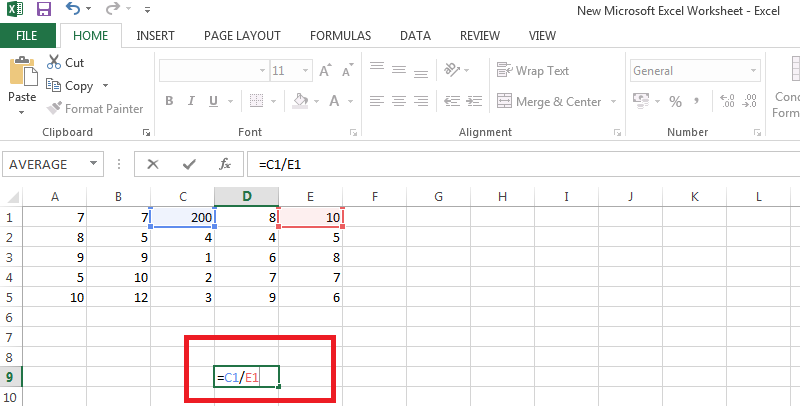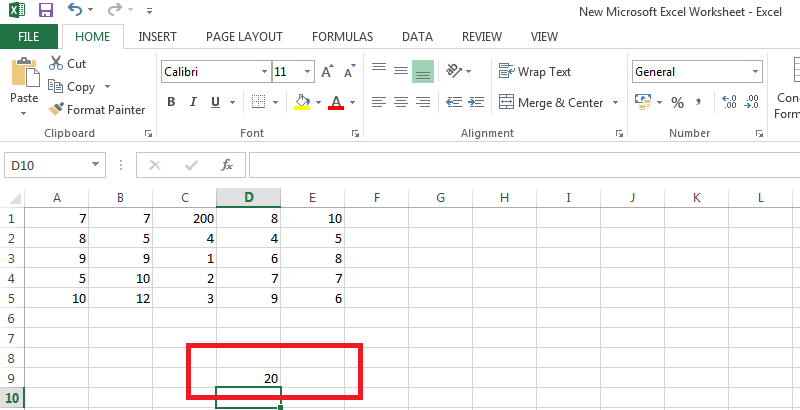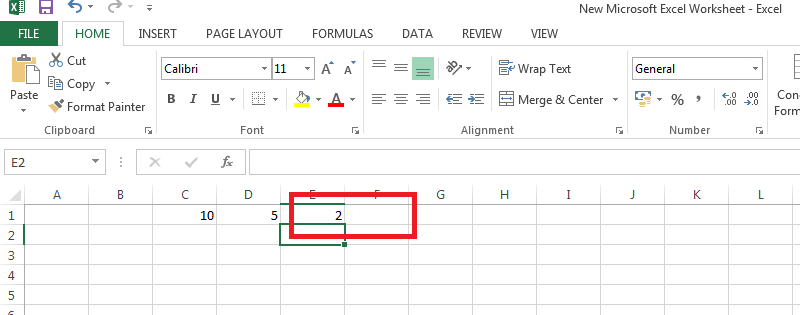Schemaninja# How to Divide in Excel?

Affiliate disclosure: In full transparency – some of the links on our website are affiliate links, if you use them to make a purchase we will earn a commission at no additional cost for you (none whatsoever!).

There is no denying the fact that Microsoft Excel is the most important computer software program used in the workplace today. Working on Excel can be a formidable task if you are not too familiar with Excel formulas.

Whether it is how to divide in Excel or multiplication or addition, the work becomes easier when you use a formula. People are intimidated by formulas, but to their surprise, Excel formulas comprise of simple tricks and a few rules that are easier to learn.

Once you become well- versed with the formulas tasks like division in excel, how to multiply, how to subtract and how to add within your spreadsheet becomes facile.

The addition is a plus sign (+), multiplication is an asterisk (*), subtraction is a minus sign (–) and division is a slash (/). Let’s throw some light on this and study the rules in detail:

# Formula and its Rules

A formula is an expression which calculates the value of a cell. They are equations that make things happen in Excel. These formulas are one of the reasons why Excel is so powerful. Some basic formula rules that you need to abide by:

• Formulas in Excel always begin with the equal sign ( = )
• The equal sign always goes in the cell where you want the answer to go
• The division symbol is the forward slash ( / )
• Cells are identified by their column letter followed by their row number, so B1 or G8, for example.
• The formula is completed by pressing the Enter key on the keyboard

For example, if you type =10/5 in a cell, the cell displays 2.

## Steps on How to Divide in Excel

Division in Excel is a very simple process and involves the use of a very easy formula. You will be amazed by how learning these formulas not only enhances your productivity but also helps you to save a lot of time. Following are the steps you need to kingpin upon for division formula in excel:

1. Open a new workbook in Excel by going to File–>New. In case you want to use an existing work book, open it by going to File–>Open.
2. Figure out which cells you would like to divide.
3. Now, type the formula directly into the cell, or type it in the formula bar at the top of your screen.
4. Divide cells C1 and E1, let’s say which is dividing 200 by 10. The equation looks like this: =C1/E15. Click away from the cell or press enter.
6. You will see the number 20 appear, which, is the product of 200/10.Note- Be sure to type an equal sign (=) in the cell before you type the numbers otherwise, Excel will interpret what you type as a date. For example, if you type 6/30, Excel may display 30-June in the cell.

### Divide numbers by using cell references

A cell reference combines the column letter and row numbers, such as A1 or F345.  The main benefit of using cell references in a formula instead of the cell value is that you can change the value without having to modify the formula.

1. Type numbers in cells C1 and D1.
For example, type 10 and 5.
2. In cell E1, type an equal sign (=) to start the formula.
3. After the equal sign, type C1/D1.4. Press RETURN.
5. If you use the example numbers, the result is 2.In case you change the value of C1 or D1 and then press RETURN, the value of E1 will undergo a change without hampering the formula. In short, there will be no change in the formula. In case you wanna add columns in Excel, here is some insightful information for you.

### Dividing through Pointing

Pointing involves clicking on the cell containing the data with the mouse pointer to add the cell reference to the formula.

To enter the excel formula for division through the pointing method:

1. Type an equal sign in a cell to begin the formula
2. Click on another cell with the mouse pointer to add that cell reference to the formula after the equal sign
3. Type the division sign – the forward slash – ( / ) into the cell you want to divide after the cell reference
4. Press the Enter key on the keyboard to complete the formula
5. The answer should be present in the cell with the division sign
6. You will be able to see the formula in the formula bar above the worksheet.

### Percentages through Division Formulas

The term “percent” is derived from the Latin per centum, meaning “by the hundred.” A percentage is a fraction that is calculated by dividing the numerator by the denominator and multiplying the result by 100.

The general form of the equation is:

= (numerator/denominator)*100

For example, if you had 20 pens and you gave 5 to your sister, how much did you give, percentage wise? It will be like this =5/20*100 you get the answer – 25%.

Excel has a very easy technique for the percentages. Just change the formatting in the cell to the percent formatting. Once percent formatting is applied in Excel, the program multiplies the decimal value by 100 and adds the percent symbol.

### Errors Reported

The most common error associated with excel division formula is the #DIV/0! Error Value. This error occurs when the denominator in the division formula is equal to zero. In short, it is produced when you attempt to divide by zero.

The main reason for this particular error is that the formula was found with an incorrect cell reference. Thus, the changing cell references result in the error.

In order to avoid this error, make sure the divisor in the function or formula isn’t zero or a blank cell. Another option can be to change the cell reference in the formula to another cell that doesn’t have a blank value.

#### Conclusion

Excel comprises of endless formulas to make your task easier. They may seem complex initially, but once you practice them, you tend to realize that they are nothing but just a piece of cake. You can do a lot of great stuff on MS Excel to make your data in best presentable form. One of the way to make your data appealing is Charts. Here is the guide to Create Charts in MS Excel.

How to divide in excel involves fabricating a formula, as there is no DIVIDE function in Excel.

Goof around with different formulas and you will be surprised each time with your new accomplishment. Follow various online tutorials to learn those complex formulas and be a shining star in your workplace!##### arun singh

Arun Singh is a experienced server management geek and he has more than 8 years of experienced handling hosting server and he do sometimes share his knowledge in digital marketing. He currently with private company in Mumbai, India and he handles server management at BloggersIdeas.com.